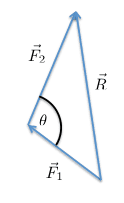# Force Resultants

I just wanna know the difference between those rules:

1. R^2 = F1^2 * F2^2 + 2*F1*F2*COS(the angle between F1 and F2)

2. The second is about the parallelogram rule, it says that the two vectors are added and their summation is the magnitude of the resultant.

Which one is correct?

tnich
Homework Helper
I just wanna know the difference between those rules:

1. R^2 = F1^2 * F2^2 + 2*F1*F2*COS(the angle between F1 and F2)

2. The second is about the parallelogram rule, it says that the two vectors are added and their summation is the magnitude of the resultant.

Which one is correct?
If I correctly understand you, both are correct. You seem to have the sign wrong in the equation. ##R^2=F_1^2+F_2^2-2F_1F_2\cos(\theta)##

Last edited:
tnich
Homework Helper
If I correctly understand you, both are correct. You seen to have the sign wrong in the equation. ##R^2=F_1^2+F_2^2-2F_1F_2\cos(\theta)##
Here is a diagram showing my understanding of the problem.#### Attachments

Orodruin
Staff Emeritus
Homework Helper
Gold Member
2021 Award
Here is a diagram showing my understanding of the problem.
View attachment 230677
That would typically not be ”the angle between the forces”. The angle between two forces in the same direction would typically be zero, whereas your convention would be pi.

tnich
Homework Helper
That would typically not be ”the angle between the forces”. The angle between two forces in the same direction would typically be zero, whereas your convention would be pi.
I agree. I am trying to interpret what the OP has written.

robphy
Homework Helper
Gold Member
Rule 1 is the dot-product [law of cosines] (which is a metrical statement).
Note that the angle-between-the-vectors (tails together, as in the parallelogram method of addition) is not the interior angle in the triangle (in the tail-to-tip method of addition).

The parallelogram rule for adding vectors is true, independent of the metric.
That tells you how to add two vectors... with the tails together, construct a parallelogram, and draw from the common tail to the opposite corner.
That's the resultant vector.
Getting the magnitude of the vector-sum is a different step [see rule 1].

sophiecentaur•